•5星
5.03MB guoruibin123 2021-08-05 17:09:37
•5星
323KB guoruibin123 2021-06-24 15:21:31
•5星
257KB weixin_42696271 2021-09-11 12:52:28
•5星
2KB GJZGRB 2021-02-19 15:22:54
•5星
178.06MB guoruibin123 2021-06-25 13:54:42
•5星
50KB didiaopao 2021-07-30 17:43:04
•5星
4.9MB KOBEYU652453 2021-08-15 10:29:03
•5星
599KB GZXGYZ 2021-04-12 16:39:55
•5星
2.58MB alongiii 2021-01-04 10:37:10
• 基于FPGA实现CIC升采样滤波器verilog HDL代码总结 FPGA 升采样

基于FPGA实现CIC升采样滤波器verilog HDL代码总结一、概述二、设计说明三、代码实现1、顶层代码2、产生正弦信号代码（调用了xilinx RAM IP）3、CIC升采样滤波实现代码四、仿真结果 一、概述 上文中我们总结了CIC基本...

一、概述

上文中我们总结了CIC基本原理设计，本文将基于xilinx FPGA通过verilog HDL实现CIC升采样及仿真情况总结如下，作为相关项目开发参考。

二、设计说明

系统工作时钟50M
正弦信号频率50M/16/8=0.390625MHz
正弦信号采样频率50M/16=3.125Msps，每个正弦波周期采集8个点
正弦信号升采样频率50M/2=25Msps，即8倍内插
CIC升采样滤波器实现框图及内部信号定义：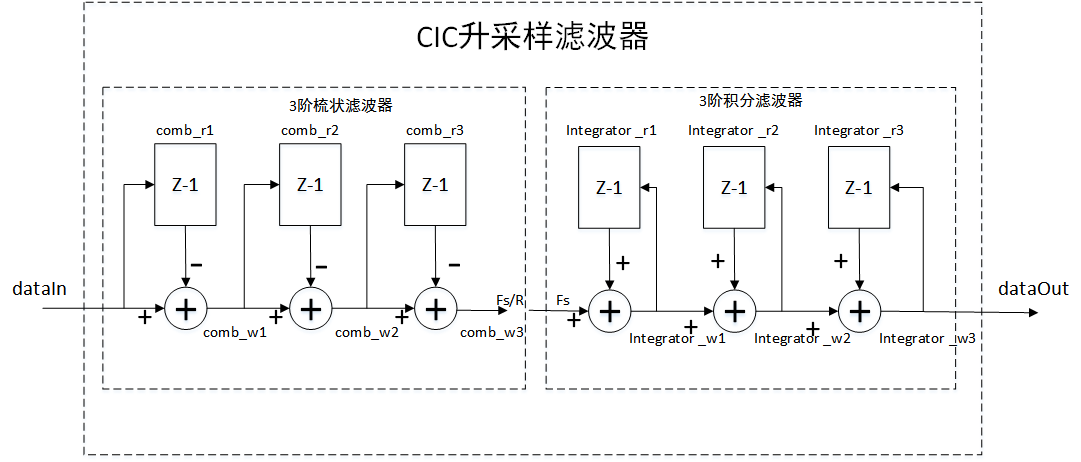三、代码实现

1、顶层代码

module top(
input				wire					sclk,
input				wire					rst_n,
input				wire	[31:0]	        phase_init,
input				wire	[31:0]          freq_para,
output              wire	[23:0]		    dataout
);

wire [7:0] sin_data;
wire data_valib;

pro_sin pro_sin_inst(
.sclk      (sclk), //系统时钟50M
.rst_n     (rst_n),
.phase_init(phase_init),//初始相位
.freq_para (freq_para),//频率配置字
.data_v    (data_valib),
.dataout   (sin_data)
);

cic_filter cic_filter_inst(
.sclk    (sclk),    //系统频率为50M
.rst_n   (rst_n),
.datain  (sin_data),
.data_v  (data_valib),
.dataout (dataout)
);
endmodule

2、产生正弦信号代码（调用了xilinx RAM IP）

module pro_sin(
input				wire						sclk, //系统时钟50M
input				wire						rst_n,
input				wire 		[31:0]	        phase_init,//初始相位
input				wire		[31:0]          freq_para,//频率配置字
output              reg                         data_v,//输出有效标识
output			    wire		[7:0]		     dataout
);

reg  [31:0] phase;//相位累加器

parameter  DIV=16-1; //分频系数，用于产生采样频率50M/16=3.125Msps的正弦信号
reg  [5:0] div_cnt;//分频计数器
reg		   div_flag;

always@(posedge sclk or negedge rst_n)
begin
if(rst_n==1'b0)
div_cnt<=6'd0;
else if(div_cnt==DIV)
div_cnt<=6'd0;
else
div_cnt<=div_cnt+1'b1;
end

always@(posedge sclk or negedge rst_n)
begin
if(rst_n==1'b0)
div_flag<=1'b0;
else if(div_cnt==6'd0)
div_flag<=1'b1;
else
div_flag<=1'b0;
end

always@(posedge sclk or negedge rst_n)
begin
if(rst_n==1'b0)
phase<=phase_init;
else if(div_flag==1'b1)
phase<=phase+freq_para;	//频率配置字由f1=M*fs/2^N计算得到M值。
end

always@(posedge sclk or negedge rst_n)
begin
if(rst_n==1'b0)
data_v<=1'b0;
else
data_v<=1'b1;
end

blk_mem_gen_0 blk_mem_gen_0_inst (
.clka(sclk),    // input wire clka
.ena(1'b1),      // input wire ena
.wea(1'b0),      // input wire [0 : 0] wea
.dina('d0),     // input wire [7 : 0] dina
.douta(dataout)  // output wire [7 : 0] douta
);

endmodule

3、CIC升采样滤波实现代码

module cic_filter(
input					wire				sclk,    //系统频率为50M
input					wire				rst_n,   //复位信号
input					wire	[7:0]	    datain,  //DDS输入的8为正弦波信号
input					wire	            data_v,  //输入信号的有效标识
output				    wire	[23:0]      dataout
);

parameter DIV=16-1; //分频系数，信号采样频率：50M/16=3.125Msps
parameter DIV_R=2-1;//分频系数，8倍内插后的信号采样频率：50M/2=25Msps
parameter W=24;//8倍内插后的信号位宽

reg [5:0] div_cnt;   //原采样率分频计数器
reg [4:0] div_cnt_r; //内插后采样率分频计数器
reg       div_flag;  //16倍分频标识
reg       div_flag_r;//8倍内插后的分频标识
//梳妆滤波器寄存器
reg [W-1:0]  comb1_r;
reg [W-1:0]  comb2_r;
reg [W-1:0]  comb3_r;
wire [W-1:0]  comb1_w;
wire [W-1:0]  comb2_w;
wire [W-1:0]  comb3_w;
//积分器寄存器
reg [W-1:0]  integrator1_r;
reg [W-1:0]  integrator2_r;
reg [W-1:0]  integrator3_r;
wire [W-1:0]  integrator1_w;
wire [W-1:0]  integrator2_w;
wire [W-1:0]  integrator3_w;

//对50M系统时钟进行16分频
always@(posedge sclk or negedge rst_n)
begin
if(rst_n==1'b0)
div_cnt<=6'd0;
else if(div_cnt==DIV)
div_cnt<=6'd0;
else
div_cnt<=div_cnt+1'b1;
end

always@(posedge sclk or negedge rst_n)
begin
if(rst_n==1'b0)
div_flag<=1'b0;
else if(div_cnt==6'd0)
div_flag<=1'b1;
else
div_flag<=1'b0;
end
//对50M系统时钟进行2分频
always@(posedge sclk or negedge rst_n)
begin
if(rst_n==1'b0)
div_cnt_r<=5'd0;
else if(div_cnt_r==DIV_R)
div_cnt_r<=5'd0;
else
div_cnt_r<=div_cnt_r+1'b1;
end

always@(posedge sclk or negedge rst_n)
begin
if(rst_n==1'b0)
div_flag_r<=1'b0;
else if(div_cnt_r==5'd0)
div_flag_r<=1'b1;
else
div_flag_r<=1'b0;
end
//梳妆滤波器
assign  comb1_w={{16{datain}},datain}-comb1_r;
assign  comb2_w=comb1_w-comb2_r;
assign  comb3_w=comb2_w-comb3_r;

always@(posedge sclk or negedge rst_n)
begin
if(rst_n==1'b0)
begin
comb1_r<='d0;
comb2_r<='d0;
comb3_r<='d0;
end
else if((data_v==1'b1)&&(div_flag==1'b1))
begin
comb1_r<={{16{datain}},datain};
comb2_r<=comb1_w;
comb3_r<=comb2_w;
end
else if(data_v==1'b0)
begin
comb1_r<='d0;
comb2_r<='d0;
comb3_r<='d0;
end
end
//积分器
assign  integrator1_w=comb3_w+integrator1_r;
assign  integrator2_w=integrator1_w+integrator2_r;
assign  integrator3_w=integrator2_w+integrator3_r;

always@(posedge sclk or negedge rst_n)
begin
if(rst_n==1'b0)
begin
integrator1_r<='d0;
integrator2_r<='d0;
integrator3_r<='d0;
end
else if((data_v==1'b1)&&(div_flag_r==1'b1))
begin
integrator1_r<=integrator1_w;
integrator2_r<=integrator2_w;
integrator3_r<=integrator3_w;
end
end
assign dataout=integrator3_w;

endmodule

四、仿真结果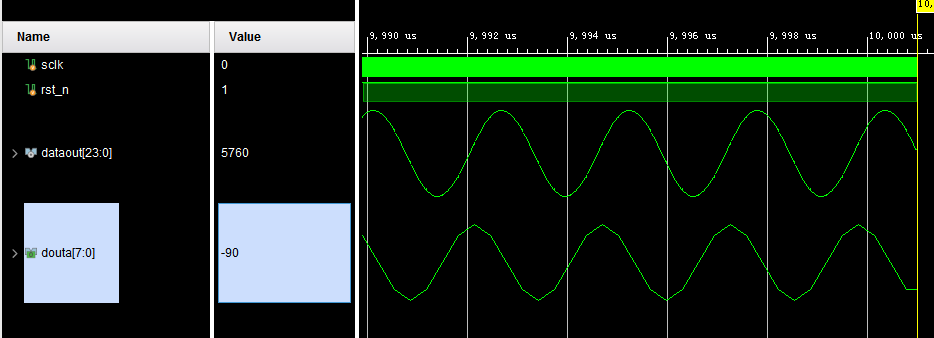展开全文weixin_39789553 2021-10-14 11:07:16
• 升、降采样的滤波器组实现形式 升采样 数字信号处理 滤波器组

升采样滤波器组实现形式引言具体推导记号推导直接实现方式滤波器组实现方式拆分信号重构信号 引言 现代通信系统的收发机部分已经广泛地采用数字信号处理技术，实现信号处理。而实现这种替代的首要问题便是将之前...

引言

现代通信系统的收发机部分已经广泛地采用数字信号处理技术，实现信号处理。而实现这种替代的首要问题便是将之前的调制，滤波等技术变为数字处理。滤波的数字处理时通过设计FIR或IIR滤波器实现的，其设计方法是《数字信号处理》这门课程的重点之一，再次不加赘述。然而使用滤波器解决的实际问题便是匹配滤波。匹配滤波要求将一个方形的码元变化成升余弦型，而方形的码元，为了简便起见，在前序处理中是用单值表示的，但升余弦型码元肯定需要更多的值来描述，因此这里涉及到了高采样率的滤波器通过一个低采样率的信号的问题。对于这种实际问题，我们可以采用多采样率信号处理技术，也即引入“升/降采样”，对信号进行处理。
然而，在数字系统中，我们首先希望降低运算量，提高系统的效率，我们发现使用升采样的系统，如果采用直接实现方式，升采样中大量的0将会参与计算，这将提升计算的冗余度，因此，从公式推导中我们发现了一种低运算量的实现方式。

升采样具体推导

记号

为了区分不同采样率的信号，我们需要在信号右下角给出采样间隔：
原始采样间隔： T T ,升采样采样间隔： T L T_{L} ,满足： T = L T L T=LT_{L}
信号 s [ n ] L s[n]_{L} 为原始采样速率的信号，而 s [ n ] 1 s[n]_{1} 代表升采样后的信号。

推导

直接实现方式

c [ n ] 1 c[n]_{1} 为处理完成的信号，它由 s [ n ] L s[n]_{L} 经过了如下处理：

1. 以按整数因子L升采样得到信号 s [ n ] 1 s[n]_{1} ,并有关系： s [ n L ] 1 = s [ n ] L s[nL]_{1}=s[n]_{L} ，且当 n ≠ k L ， k ∈ Z n\neq kL，k\in Z 时， s [ n ] 1 = 0 s[n]_{1}=0
2. 通过低通滤波器 g t x [ n ] 1 g_{tx}[n]_{1} ，使得波形更加连续，得到信号 c [ n ] 1 c[n]_{1}

则信号通过滤波器可以写作如下卷积：
c [ n ] 1 = s [ n ] 1 ∗ g t x [ n ] 1 = ∑ m = − ∞ + ∞ s [ m ] 1 g t x [ n − m ] 1 = ∑ k L = − ∞ + ∞ s [ k L ] 1 g t x [ n − k L ] 1 = ∑ k = − ∞ + ∞ s [ k ] L g t x [ n − k L ] 1 \begin{aligned} c[n]_{1} &amp;= s[n]_{1}*g_{tx}[n]_{1} \\ &amp;=\sum^{+\infty}_{m=-\infty}s[m]_{1}g_{tx}[n-m]_{1}\\ &amp;=\sum^{+\infty}_{kL=-\infty}s[kL]_{1}g_{tx}[n-kL]_{1}\\ &amp;=\sum^{+\infty}_{k=-\infty}s[k]_{L}g_{tx}[n-kL]_{1} \end{aligned}

滤波器组实现方式

拆分信号

我们希望把最后一个式子写作两个原始采样率的信号的卷积，于是有了下面的记号：
记 c l [ n ] L = c [ n L + l ] 1 l = 0 , 1 , ⋯ &ThinSpace; , L − 1 记 g t x l [ n ] L = g t x [ n L + l ] 1 l = 0 , 1 , ⋯ &ThinSpace; , L − 1 c l [ n ] L = ∑ k = − ∞ + ∞ s [ k ] L g t x [ ( n L + l ) − k L ] 1 = ∑ k = − ∞ + ∞ s [ k ] L g t x [ ( n − k ) L + l ] 1 = ∑ k = − ∞ + ∞ s [ k ] L g t x l [ n − k ] L \begin{aligned} \text{记}c^{l}[n]_{L}&amp;=c[nL+l]_{1}\quad l=0,1,\cdots,L-1\\ \text{记}g_{tx}^{l}[n]_{L}&amp;=g_{tx}[nL+l]_{1}\quad l=0,1,\cdots,L-1\\ c^{l}[n]_{L}&amp;=\sum^{+\infty}_{k=-\infty}s[k]_{L}g_{tx}[(nL+l)-kL]_{1}\\ &amp;=\sum^{+\infty}_{k=-\infty}s[k]_{L}g_{tx}[(n-k)L+l]_{1}\\ &amp;=\sum^{+\infty}_{k=-\infty}s[k]_{L}g_{tx}^{l}[n-k]_{L}\\ \end{aligned}
这样就得到了 c [ n ] 1 c[n]_{1} 中的L个子集，这些子集是由低采样率的信号卷积组成的。接下来的任务是找到L个子集拼接成 c [ n ] 1 c[n]_{1} 的方法

重构信号

先对信号进行增采样：
记 c l [ n ] 1 = ∑ m = − ∞ + ∞ c l [ m ] L δ [ n − m L ] 1 l = 0 , 1 , ⋯ &ThinSpace; , L − 1 \text{记}c^{l}[n]_{1}=\sum^{+\infty}_{m=-\infty}c^{l}[m]_{L}\delta[n-mL]_{1}\quad l=0,1,\cdots,L-1
我们用矩阵的形式写出信号的表达式，假设L=5：
[ c 0 [ n ] 1 c 1 [ n ] 1 c 2 [ n ] 1 c 3 [ n ] 1 c 4 [ n ] 1 ] = [ ⋯ c 0 [ 0 ] L 0 0 0 0 c 0 [ 1 ] L 0 0 0 0 ⋯ ⋯ c 1 [ 0 ] L 0 0 0 0 c 1 [ 1 ] L 0 0 0 0 ⋯ ⋯ c 2 [ 0 ] L 0 0 0 0 c 2 [ 1 ] L 0 0 0 0 ⋯ ⋯ c 3 [ 0 ] L 0 0 0 0 c 3 [ 1 ] L 0 0 0 0 ⋯ ⋯ c 4 [ 0 ] L 0 0 0 0 c 4 [ 1 ] L 0 0 0 0 ⋯ ] \left[ \begin{matrix} c^{0}[n]_{1}\\ c^{1}[n]_{1}\\ c^{2}[n]_{1}\\ c^{3}[n]_{1}\\ c^{4}[n]_{1}\\ \end{matrix} \right]= \left[ \begin{matrix} \cdots&amp;c^{0}_{L}&amp;0&amp;0&amp;0&amp;0&amp;c^{0}_{L}&amp;0&amp;0&amp;0&amp;0\cdots\\ \cdots&amp;c^{1}_{L}&amp;0&amp;0&amp;0&amp;0&amp;c^{1}_{L}&amp;0&amp;0&amp;0&amp;0\cdots\\ \cdots&amp;c^{2}_{L}&amp;0&amp;0&amp;0&amp;0&amp;c^{2}_{L}&amp;0&amp;0&amp;0&amp;0\cdots\\ \cdots&amp;c^{3}_{L}&amp;0&amp;0&amp;0&amp;0&amp;c^{3}_{L}&amp;0&amp;0&amp;0&amp;0\cdots\\ \cdots&amp;c^{4}_{L}&amp;0&amp;0&amp;0&amp;0&amp;c^{4}_{L}&amp;0&amp;0&amp;0&amp;0\cdots\\ \end{matrix} \right]
接着进行移位：
[ c 0 [ n − 0 ] 1 c 1 [ n − 1 ] 1 c 2 [ n − 2 ] 1 c 3 [ n − 3 ] 1 c 4 [ n − 4 ] 1 ] = [ ⋯ c 0 [ 0 ] L 0 0 0 0 c 0 [ 1 ] L 0 0 0 0 ⋯ ⋯ 0 c 1 [ 0 ] L 0 0 0 0 c 1 [ 1 ] L 0 0 0 ⋯ ⋯ 0 0 c 2 [ 0 ] L 0 0 0 0 c 2 [ 1 ] L 0 0 ⋯ ⋯ 0 0 0 c 3 [ 0 ] L 0 0 0 0 c 3 [ 1 ] L 0 ⋯ ⋯ 0 0 0 0 c 4 [ 0 ] L 0 0 0 0 c 4 [ 1 ] L ⋯ ] = [ ⋯ c [ 0 ] 1 0 0 0 0 c [ 5 ] 1 0 0 0 0 ⋯ ⋯ 0 c [ 1 ] 1 0 0 0 0 c [ 6 ] 1 0 0 0 ⋯ ⋯ 0 0 c [ 2 ] 2 0 0 0 0 c [ 7 ] 1 0 0 ⋯ ⋯ 0 0 0 c [ 3 ] 1 0 0 0 0 c [ 8 ] 1 0 ⋯ ⋯ 0 0 0 0 c [ 4 ] 1 0 0 0 0 c [ 9 ] 1 ⋯ ] \begin{aligned} \left[ \begin{matrix} c^{0}[n-0]_{1}\\ c^{1}[n-1]_{1}\\ c^{2}[n-2]_{1}\\ c^{3}[n-3]_{1}\\ c^{4}[n-4]_{1}\\ \end{matrix} \right]&amp;= \left[ \begin{matrix} \cdots&amp;c^{0}_{L}&amp;0&amp;0&amp;0&amp;0&amp;c^{0}_{L}&amp;0&amp;0&amp;0&amp;0&amp;\cdots\\ \cdots&amp;0&amp;c^{1}_{L}&amp;0&amp;0&amp;0&amp;0&amp;c^{1}_{L}&amp;0&amp;0&amp;0&amp;\cdots\\ \cdots&amp;0&amp;0&amp;c^{2}_{L}&amp;0&amp;0&amp;0&amp;0&amp;c^{2}_{L}&amp;0&amp;0&amp;\cdots\\ \cdots&amp;0&amp;0&amp;0&amp;c^{3}_{L}&amp;0&amp;0&amp;0&amp;0&amp;c^{3}_{L}&amp;0&amp;\cdots\\ \cdots&amp;0&amp;0&amp;0&amp;0&amp;c^{4}_{L}&amp;0&amp;0&amp;0&amp;0&amp;c^{4}_{L}&amp;\cdots\\ \end{matrix} \right]\\&amp;= \left[ \begin{matrix} \cdots&amp;c_{1}&amp;0&amp;0&amp;0&amp;0&amp;c_{1}&amp;0&amp;0&amp;0&amp;0&amp;\cdots\\ \cdots&amp;0&amp;c_{1}&amp;0&amp;0&amp;0&amp;0&amp;c_{1}&amp;0&amp;0&amp;0&amp;\cdots\\ \cdots&amp;0&amp;0&amp;c_{2}&amp;0&amp;0&amp;0&amp;0&amp;c_{1}&amp;0&amp;0&amp;\cdots\\ \cdots&amp;0&amp;0&amp;0&amp;c_{1}&amp;0&amp;0&amp;0&amp;0&amp;c_{1}&amp;0&amp;\cdots\\ \cdots&amp;0&amp;0&amp;0&amp;0&amp;c_{1}&amp;0&amp;0&amp;0&amp;0&amp;c_{1}&amp;\cdots\\ \end{matrix} \right] \end{aligned}
于是有：
c [ n ] 1 = ∑ l = 0 L − 1 c l [ n − l ] 1 c[n]_{1}=\sum^{L-1}_{l=0}c^{l}[n-l]_{1}
将表达式整合即有：
c [ n ] 1 = ∑ l = 0 L − 1 c l [ n − l ] 1 = ∑ l = 0 L − 1 ∑ m = − ∞ + ∞ c l [ m ] L δ [ n − m L − l ] 1 = ∑ l = 0 L − 1 ∑ m = − ∞ + ∞ ( ∑ k = − ∞ + ∞ s [ k ] L g t x l [ n − k ] L ) δ [ n − m L − l ] 1 \begin{aligned} c[n]_{1}&amp;=\sum^{L-1}_{l=0}c^{l}[n-l]_{1}\\ &amp;=\sum^{L-1}_{l=0}\sum^{+\infty}_{m=-\infty}c^{l}[m]_{L}\delta[n-mL-l]_{1}\\ &amp;=\sum^{L-1}_{l=0}\sum^{+\infty}_{m=-\infty}(\sum^{+\infty}_{k=-\infty}s[k]_{L}g_{tx}^{l}[n-k]_{L})\delta[n-mL-l]_{1} \end{aligned}
用滤波器组实现升采样的步骤可以归纳为：

1. 将原始采样速率的信号分别通过 g t x l [ n − k ] L g_{tx}^{l}[n-k]_{L} 得到n组信号 c l [ n ] L c^{l}[n]_{L}
2. 将n组信号分别进行升采样，得到 c l [ n ] 1 c^{l}[n]_{1}
3. 将不同的信号流按标号延迟，并相加得到原信号 c [ n ] 1 c[n]_{1}

降采样具体推导

记号

为了区分不同采样率的信号，我们需要在信号右下角给出采样间隔：
原始采样间隔： T T ,降采样采样间隔： T M T_{M} ,满足： T M = M T T_{M}=MT
信号 s [ n ] 1 s[n]_{1} 为原始采样速率的信号，而 s [ n ] M s[n]_{M} 代表升采样后的信号。

推导

直接实现方式

y [ n ] M y[n]_{M} 为处理完成的信号，它由 z [ n ] 1 z[n]_{1} 经过了如下处理：

1. 通过低通滤波器 g r x [ n ] 1 g_{rx}[n]_{1} ，滤去多余信号，得到信号 y [ n ] 1 y[n]_{1}
2. 以按整数因子M降采样得到信号 y [ n ] M y[n]_{M} ,并有关系： y [ n ] M = y [ n M ] 1 y[n]_{M}=y[nM]_{1}

则信号通过滤波器可以写作如下卷积：
y [ n ] M = ∑ m = − ∞ + ∞ z [ m ] 1 g r x [ n M − m ] 1 = ∑ m = − ∞ + ∞ z [ n M − m ] 1 g r x [ m ] 1 \begin{aligned} y[n]_{M} &amp;=\sum^{+\infty}_{m=-\infty}z[m]_{1}g_{rx}[nM-m]_{1}\\ &amp;=\sum^{+\infty}_{m=-\infty}z[nM-m]_{1}g_{rx}[m]_{1}\\ \end{aligned}

滤波器组实现方式

拆分信号

我们希望把最后一个式子写作两个原始采样率的信号的卷积，进行变形：
令 m = p M − k y [ n ] M = ∑ m = − ∞ + ∞ z [ m ] 1 g r x [ n M − m ] 1 = ∑ p = − ∞ + ∞ ∑ k = 0 M − 1 z [ p M − k ] 1 g r x [ n M − p M + k ] 1 = ∑ k = 0 M − 1 ∑ p = − ∞ + ∞ z [ p M − k ] 1 g r x [ n M − p M + k ] 1 \begin{aligned} \text{令}m=pM-k\\ y[n]_{M} &amp;=\sum^{+\infty}_{m=-\infty}z[m]_{1}g_{rx}[nM-m]_{1}\\ &amp;=\sum^{+\infty}_{p=-\infty}\sum^{M-1}_{k=0}z[pM-k]_{1}g_{rx}[nM-pM+k]_{1}\\ &amp;=\sum^{M-1}_{k=0}\sum^{+\infty}_{p=-\infty}z[pM-k]_{1}g_{rx}[nM-pM+k]_{1}\\ \end{aligned}
如果：
记 z k [ p ] M = z [ p M − k ] 1 k = 0 , 1 , ⋯ &ThinSpace; , M − 1 记 g r x k [ m ] M = g r x [ m M + k ] 1 k = 0 , 1 , ⋯ &ThinSpace; , M − 1 y [ n ] M = ∑ k = 0 M − 1 ∑ p = − ∞ + ∞ z [ p M − k ] 1 g r x [ n M − p M + k ] 1 = ∑ k = 0 M − 1 ∑ p = − ∞ + ∞ z k [ p ] M g r x k [ n − p ] M = ∑ k = 0 M − 1 z k [ n ] M ∗ g r x k [ n ] M \begin{aligned} \text{记}z^{k}[p]_{M}&amp;=z[pM-k]_{1}\quad k=0,1,\cdots,M-1\\ \text{记}g_{rx}^{k}[m]_{M}&amp;=g_{rx}[mM+k]_{1}\quad k=0,1,\cdots,M-1\\ y[n]_{M} &amp;=\sum^{M-1}_{k=0}\sum^{+\infty}_{p=-\infty}z[pM-k]_{1}g_{rx}[nM-pM+k]_{1}\\ &amp;=\sum^{M-1}_{k=0}\sum^{+\infty}_{p=-\infty}z^{k}[p]_{M}g_{rx}^{k}[n-p]_{M}\\ &amp;=\sum^{M-1}_{k=0}z^{k}[n]_{M}*g_{rx}^{k}[n]_{M}\\ \end{aligned}
这样就得到了 y [ n ] M y[n]_{M} 的结果，可以用如下方法重构信号。

重构信号

用滤波器组实现降采样的步骤可以归纳为：

1. 将原始采样速率的信号 z [ n ] 1 z[n]_{1} 分别通过延迟器得到 z [ n − k ] 1 z[n-k]_{1} ，然后降采样得到 z [ n M − k ] 1 = z k [ n ] 1 z[nM-k]_{1}=z^{k}[n]_{1}
2. 将n组信号分别通过滤波器 g r x k [ n ] M g_{rx}^{k}[n]_{M}
3. 将所有通过滤波器的结果求和相加

滤波器拆分方法

可以看到，滤波器的拆分方法是相同的：
g t x l [ n ] L = g t x [ n L + l ] 1 l = 0 , 1 , ⋯ &ThinSpace; , L − 1 g r x k [ m ] M = g r x [ m M + k ] 1 k = 0 , 1 , ⋯ &ThinSpace; , M − 1 \begin{aligned} g_{tx}^{l}[n]_{L}&amp;=g_{tx}[nL+l]_{1}\quad l=0,1,\cdots,L-1\\ g_{rx}^{k}[m]_{M}&amp;=g_{rx}[mM+k]_{1}\quad k=0,1,\cdots,M-1\\ \end{aligned}

展开全文qq_41106156 2019-03-10 12:52:53
• focus357 2021-02-20 18:21:08
• 采样FIR滤波器的设计与硬件实现来源：luojiashao作者：华仔浏览：2051时间：2016-08-10 14:18标签：摘要：摘要：实现了一种全集成可变带宽中频宽带低通滤波器，讨论分析了跨导放大器-电容(OTA—C)连续时间型滤波器...

降采样FIR滤波器的设计与硬件实现

来源：luojiashao

作者：华仔

浏览：2051

时间：2016-08-10 14:18

标签：

摘要：

摘要：实现了一种全集成可变带宽中频宽带低通滤波器，讨论分析了跨导放大器-电容(OTA—C)连续时间型滤波器的结构、设计和具体实现，使用外部可编程电路对所设计滤波器带宽进行控制，并利用ADS软件进行电路设计和仿真验证。仿真结果表明，该滤波器带宽的可调范围为1～26 MHz，阻带抑制率大于35 dB，带内波纹小于0．5 dB，采用1．8 V电源，TSMC 0．18μm CMOS工艺库仿真，功耗小于21 mW，频响曲线接近理想状态。关键词：Butte

魏莉，林平分

(北京工业大学北京市嵌入式重点实验室，北京  100122)

0 引言

降采样数字滤波器可广泛应用于通信、声音和图像处理系统中。而当输入信号的带宽高于需处理的带宽时，对信号进行降采样处理可以大大减少数据量，提高数据率使实时处理容易实现。同时，为了克服在频域上的混叠，还需要先用低通滤波器过滤非处理带宽的信号能量，然后再降采样，以避免混叠。

本文以LTE无线通信系统为例，提出了一种完整的降采样FIR滤波器的设计和硬件实现方案。该方案在利用FDAtool得到滤波器系数之后再进行定点化，并将各系数拆分成2的幂次方相加减的形式，以便进行移位相加。对于降采样，该设计没有使用传统的先滤波后采样的方案，而是在滤波过程中渗入了采样操作。这样就大大减少了硬件资源的消耗，并可将乘法器的使用数目降低到零。

1 降采样滤波器的结构原理

降采样滤波器的典型结构如图1所示，包括抗混叠滤波器和降采样器。其中D为降采样率，k表示滤波器阶数。从图1可以看出，抗混叠滤波器的输出y(n)是对输入序列x(n)加权求和的结果，即：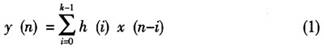降采样后的输出为：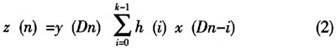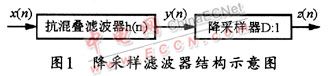直接降低采样率往往会使信号在频域上出现混叠，所以，一般需要预先通过一个低通滤波器抗混叠处理后再进行降采样，这个滤波器一般也称为抗混叠滤波器。图2所示是预滤波器的原理示意图。抗混叠滤波就是在满足一定分辨率和通信带宽的前提下，尽可能降低数据量，从而节约计算资源、节省存储空间，使实时处理容易实现。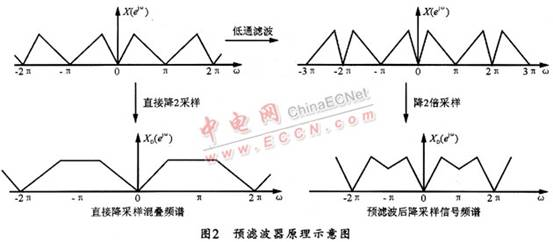2 降采样滤波器的设计与硬件实现

2．1 降采样滤波器的设计

利用matlab工具箱中自带的FDAtool可以确定滤波器的系数。首先根据系统要求确定滤波器的性能参数，比如在LTE系统中，数据传输带宽为10 MHz，其中用到一个降采样滤波器，将采样频率为61．44 MHz的信号降采样两倍后为30．72 MHz。本设计方法选择低通FIR等纹波滤波器。滤波器的阶数可以自己指定，也可以通过设置通带纹波摆幅和阻带衰减自行得到，一般通带纹波摆幅设为0．1dB，而阻带衰减设为60 dB。在满足这些性能指标的前提下，为了便于实现，滤波器的阶数设计为30阶，故有31个抽头。其设计出的低通滤波器如图3所示。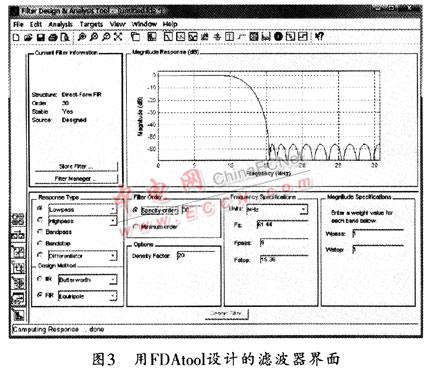单击工具栏中的[b，a]图标，就可以得到滤波器的系数。

2．2 降采样滤波器的硬件实现

根据上述方案得到滤波器系数之后，再利用FDAtool所提供的量化功能，并根据该降采样滤波器前后模块的精度需求，可以选择量化精度为12，于是得到的滤波器定点化之后的系数如下：

[b0， b1， …， b30] =[-21， -8l， -58，77，173，9，-29l，-250，284，641，33，-109l，-990，1454，4938，6599，4938，1454，-990， -1091， 33， 64l， 284， -250， -291，9，173，77，-58，-81，-21]，可见，该滤波器的系数是关于b15对称的，这一点也验证了FIR滤波器系数对称的特点。图4所示是本文初步设计的滤波器硬件架构。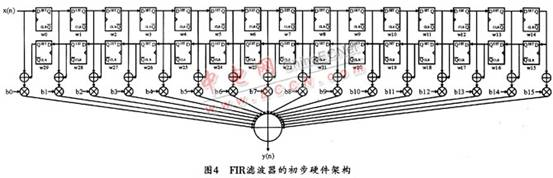为了进一步降低硬件复杂度，可将定点化的系数拆分成2的幂次方相加减的形式。以图3中设计出的系数为例：

[b0，b1，…，b15] = [-21，-8l，-58，77，173，9，-29l，-250， 284， 641， 33， -109l， -990，1454，4938，6599]

=[  -(16+4+1)，

-(64+16+1)，

-(64-8+2)，

(64+16-4+1)，

(256-64-16-4+1)，

(8+1)，

-(256+32+4-1)，

-(256-8+2)，

(256+32-4)，

(512+128+1)，

(32+1)，

-(1024+64+4-1)，

-(1024-32-2)，

(2048-512-64-16-2)，

(4096+1024-256+64+8+2)，

(8192-2048+512-64+8-1)]． (3)

结合滤波器的阶数可知式(1)中的h(n)可以表示为：

h(n)=-21×[δ(n)+δ(n-30)]-8l×[δ(n-1)+δ(n-29)]-58×[δ(n-2)+δ(n-29)]+…+6599×δ(n-15)．   (4)

于是，有：y(n)=-21×[x(n)+x(n-30)]-8l×[x(n-1)+x(n-29)]-58×[x(n-2)+x(n-29)]+…+6599×x(n-15)． (5)

将以上各系数用(3)中的拆分结果替换，可将式(5)进一步写为：

y(n)=-(16+4+1) [x(n)+x(n-30)]-(64+16+1) [x(n-1)+x(n-29)]-(64-8+2) [x(n-2)+x(n-29)]+…+(8192-2048+512-64+8-1)·x(n-15)．(6)

这样，输出y(n)可表示成输入移位相加减的形式。至此，抗混叠FIR滤波器便告设计完成。

由图2可知，抗混叠滤波器输出信号必须经过降采样才能输出。而对于本设计的滤波器，其降采样率为2：l，理论上把式(6)取出一半即可满足降采样要求，但是，这样就意味着之前浪费了一部分硬件资源去计算这一半不用的数据。基于此考虑，在设计滤波器时可加上一个降采样控制器，x(n)还是正常输入，每一拍进一个新的采样点，而滤波过程则用降采样控制器控制，这样可使计算每隔一拍进行一次。

3 仿真结果分析

3．1 滤波器的幅频响应

图5所示是在matlab中利用定点化之后的滤波器系数画出滤波器的幅频响应特性曲线。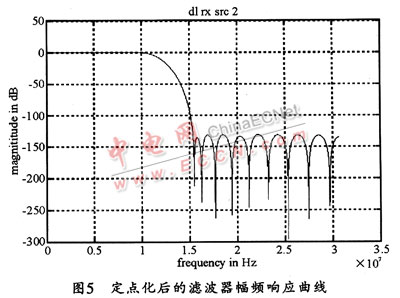该曲线与2．1中设计的滤波器幅频曲线基本一致，从而验证了本设计的正确性。

3．2 硬件代码的仿真波形

对于本文中设计的硬件架构，用Verilog语言写出相应的硬件实现代码，再用modelsim软件进行波形仿真，其仿真结果如图6所示。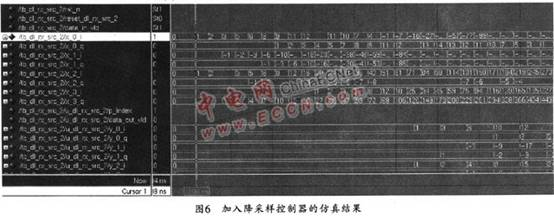由图6所示的仿真结果可见，在降采样控制器的控制下，输入滤波器的数据经过滤波器之后，其输出频率降低一半。

3．3 综合、布局和布线结果

上述设计可用QuartusⅡ9．O进行综合、布局和布线，选用stratixⅢ的器件EP3SL340F151713，所得到的硬件资源占用情况如图7所示。此时，该滤波器最高可以run到170．07 MHz，可以符合系统要求。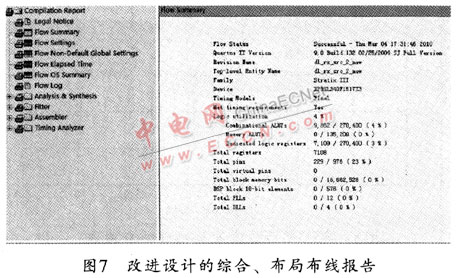实际上，用OuartusⅡ9．0也可以对传统方法设计出的滤波器进行综合、布局布线，并选择同样的器件。因为，传统的设计只是利用了系数的对称特点，而没有对系数进行分解。由于是直接和输入相乘叠加，因此滤波器的硬件代码最高只能跑到59．51MHz。其硬件资源占用情况如图8所示。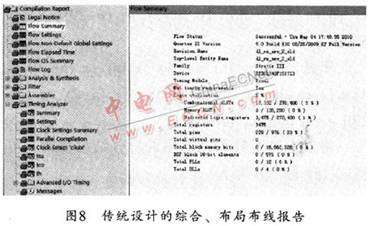比较图7和图8的报告结果可见，本文提出的设计方法在LUT资源的占有和滤波器最高工作频率方面都有明显的改进。从而解决了传统设计需要专门对滤波器输出信号进行2倍降采样而耗费硬件资源的问题。

4 结束语

本文通过基于matlab自带的工具来对降采样FIR数字滤波器进行原型设计，给出了硬件资源占用少且工作频率高的降采样滤波器的实现方案。该设计经过modelsim软件的功能仿真和QuartusⅡ软件进行综合、布局布线验证，其均可达到系统要求。

分享到:展开全文weixin_39951930 2021-04-20 08:48:01
• lovehua365 2018-03-18 11:17:52
• 11.01MB weixin_38732252 2021-06-03 16:57:47
• 【算法研究】 数字信号升采样(upsampling) 和降采样(downsampling) 技术 升采样 降采样 信号处理

qq_30404573 2019-09-03 23:09:39
• 109KB xmt1139057136 2019-03-19 12:51:52
• qq_37577735 2018-07-13 10:48:40
•5星
1KB ludonghai0417 2011-01-05 21:18:46
• Matlab中根升余弦滤波器的设置 matlab 开发语言 矩阵

alley98 2021-12-28 10:57:58
• 【滤波器】基于matlab平方根升余弦滤波器【含Matlab源码 994期】 matlab

TIQCmatlab 2021-06-13 23:36:52
• weixin_39890708 2020-12-22 13:50:59
• qq_37577735 2018-07-13 10:50:59
• paidaxingtongxue 2020-06-23 23:17:19
• 【滤波器】基于matlab升余弦滤波器【含Matlab源码 993期】 matlab

TIQCmatlab 2021-06-13 23:32:12
• matlab采样函数，上采样/下采样,插值/抽取 matlab 信号处理 数据采样

qq_23100417 2018-07-10 21:34:16
• rzld66666 2018-07-25 14:17:09
• 无码间干扰，升余弦滤波器，非线性失真 升余弦滤波器

xd15010130025 2019-03-19 14:49:07
• qq_37577735 2018-07-13 10:37:48
• li975242487 2018-06-04 11:06:13
• qq_37577735 2018-07-13 13:10:49
• 设计根升余弦滚降滤波器 matlab

qq_43466146 2021-09-29 17:59:34
• 2020.09.05降采样和升采样的理解 数字信号处理

nihaoqiaoan 2020-09-05 17:07:55
•5星
3KB FFt_serAph 2009-01-04 12:26:25...